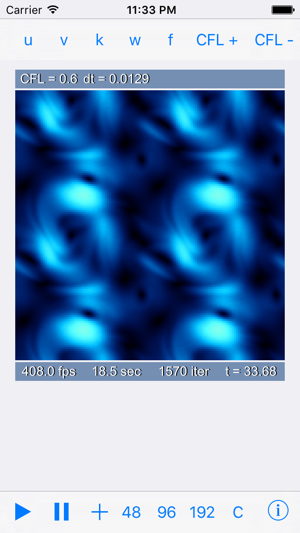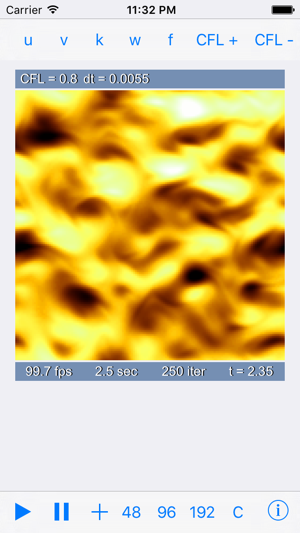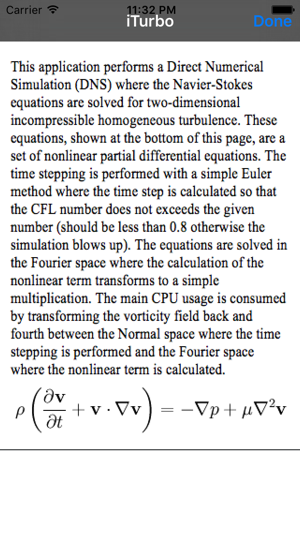## iPhone Screenshots

•••## Description

This application performs a Direct Numerical Simulation (DNS) where the Navier-Stokes equations are solved for two dimensional incompressible homogeneous turbulence. These equations are a set of nonlinear partial differential equations. The time stepping is performed with a simple Euler method where the time step is calculated so that the CFL number does not exceeds the given number (should be less than 0.8 otherwise the simulation blows up). The equations are solved in the Fourier space where the calculation of the nonlinear term transforms to a simple multiplication. The main CPU usage is consumed by transforming the vorticity field back and fourth between the Normal space where the time-stepping is performed and the Fourier space where the nonlinear term is calculated.

## What’s New

Version 1.2

Updated for iOS 7 and above

5.0 out of 5
1 Rating

1 Rating

## Information

Seller
Torbjorn Sjogren
Size
602.1 KB
Category
Education
Compatibility

Requires iOS 7.0 or later. Compatible with iPhone, iPad, and iPod touch.

Languages

English

Age Rating
4+
Price
Free

## Supports

•